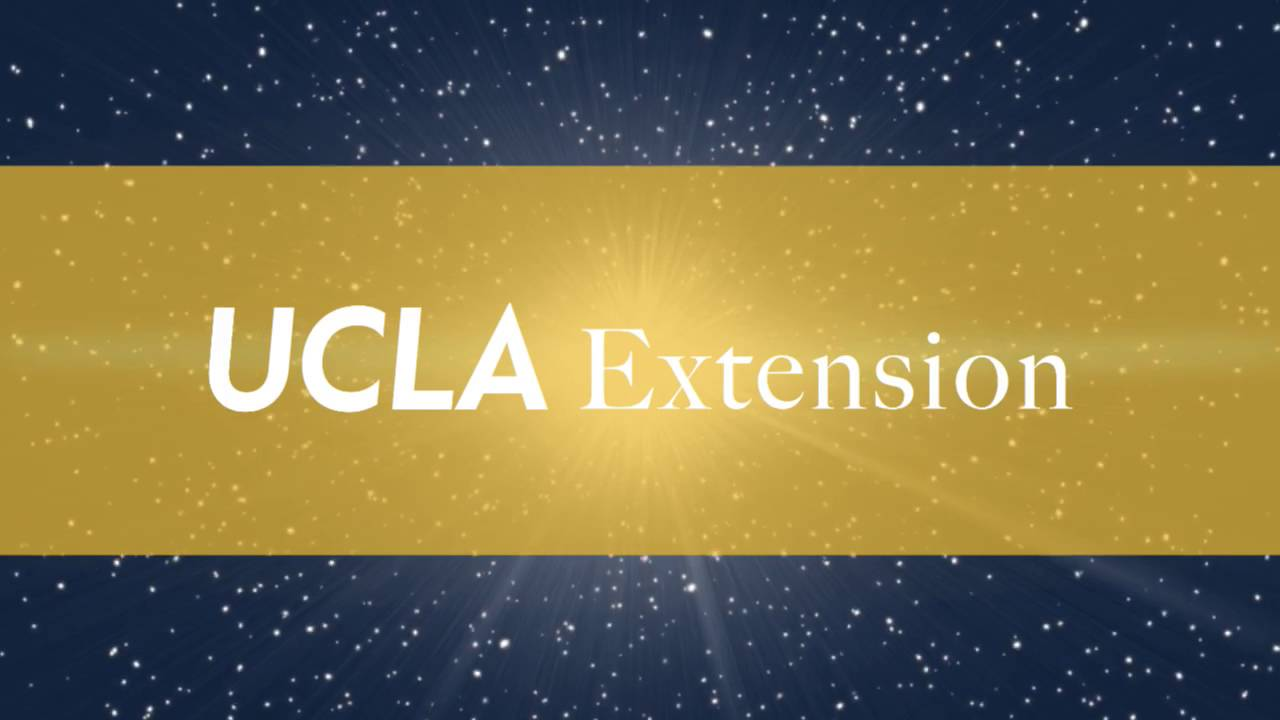# MATH X 451.43 Introduction to Algebraic Geometry: The Sequel | UCLA ExtensionBookmarked MATH X 451.43 Introduction to Algebraic Geometry: The Sequel (UCLA Extension)
Algebraic geometry is the study, using algebraic tools, of geometric objects defined as the solution sets to systems of polynomial equations in several variables. This course is the second in a two-quarter introductory sequence that develops the basic theory of this classical mathematical field. Whereas the fall-quarter course focused more on the subject’s algebraic underpinnings, this quarter will concentrate on geometric interpretations and applications. Topics to be discussed include Bézout’s Theorem, rational varieties, cubic curves and surfaces (including the remarkable 27-line theorem), and the connection between varieties and manifolds. The theoretical discussion will be supported by a large number of examples and exercises. The course should appeal to those with an interest in gaining a deeper understanding of the mathematical interplay among algebra, geometry, and topology.

Alright math nerds, it’s that time again! Be sure to register for Mike Miller’s excellent follow-on course for Algebraic Geometry.

Don’t forget to use the coupon code EARLY to save 10% with an early registration–time is limited!

Format LinkCategories Bookmark, Mathematics
Syndicated copies to: